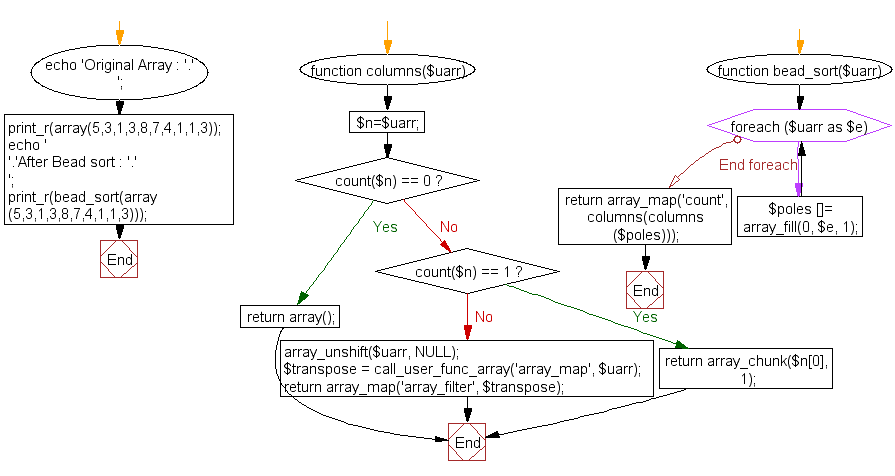﻿ PHP Array Exercise: Sort an array of positive integers using the Bead-Sort Algorithm - w3resource# PHP Array Exercises : Sort an array of positive integers using the Bead-Sort Algorithm

## PHP Array: Exercise-10 with Solution

Write a PHP program to sort an array of positive integers using the Bead-Sort Algorithm.

According to Wikipedia "Bead-sort is a natural sorting algorithm, developed by Joshua J. Arulanandham, Cristian S. Calude and Michael J. Dinneen in 2002. Both digital and analog hardware implementations of bead sort can achieve a sorting time of O(n); however, the implementation of this algorithm tends to be significantly slower in software and can only be used to sort lists of positive integers".

Input array : Array (  => 5  => 3  => 1  => 3  => 8  => 7  => 4  => 1  => 1  => 3 )

Sample Solution:

``````<?php
function columns(\$uarr)
{
\$n=\$uarr;
if (count(\$n) == 0)
return array();
else if (count(\$n) == 1)
return array_chunk(\$n, 1);
array_unshift(\$uarr, NULL);
\$transpose = call_user_func_array('array_map', \$uarr);
return array_map('array_filter', \$transpose);
}
{
foreach (\$uarr as \$e)
\$poles []= array_fill(0, \$e, 1);
return array_map('count', columns(columns(\$poles)));
}
echo 'Original Array : '.'
';
print_r(array(5,3,1,3,8,7,4,1,1,3));
echo '
';
?>
```
```

Sample Output:

```Original Array :
Array
(
 => 5
 => 3
 => 1
 => 3
 => 8
 => 7
 => 4
 => 1
 => 1
 => 3
)

Array
(
 => 8
 => 7
 => 5
 => 4
 => 3
 => 3
 => 3
 => 1
 => 1
 => 1
)
```

Flowchart:PHP Code Editor:

What is the difficulty level of this exercise?

Test your Programming skills with w3resource's quiz.

﻿

## PHP: Tips of the Day

Concatenation Operators: You can use concatenation to join strings "end to end" while outputting them (with echo or print).

You can concatenate variables using a . (period/dot).

Example:

```<?php
// String variable

\$name = 'Jhon';

// Concatenate multiple strings (3 in this example) into one and echo it once done.

echo '<p>Hello ' . \$name . ', Nice to meet you.</p>';

// Concatenation Operators
?>
```

Output:

```<p>Hello Jhon, Nice to meet you.</p>
```

Similar to concatenation, echo (when used without parentheses) can be used to combine strings and variables together (along with other arbitrary expressions) using a comma (,).

```<?php
\$itemCount = 1;
echo 'You have learn ', \$itemCount, ' Tips', \$itemCount === 1 ? '' : 's';
?>
```

Output:

```You have learn 1 Tips
```

String concatenation vs passing multiple arguments to echo

Passing multiple arguments to the echo command is more advantageous than string concatenation in some circumstances. The arguments are written to the output in the same order as they are passed in.

```echo "The total is: ", \$x + \$y;
```

The problem with the concatenation is that the period . takes precedence in the expression. If concatenated, the above expression needs extra parentheses for the correct behavior. The precedence of the period affects ternary operators too.

```echo "The total is: " . (\$x + \$y);
```